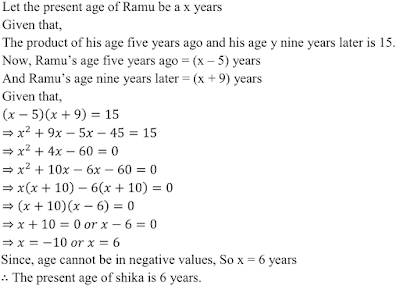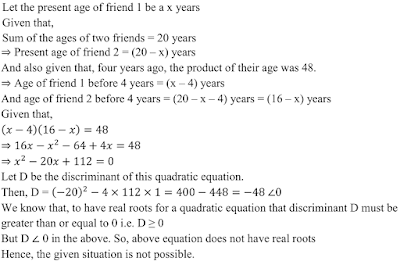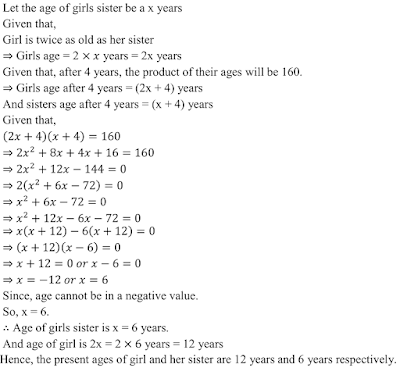>

## Chapter 8 Quadratic Equations R.D. Sharma Solutions for Class 10th Math Exercise 8.9

1. Ashu is x years old while his mother Mrs Veena is x years old. Five years hence Mrs Veena will be three times old as Ashu. Find their present ages.

Solution2. The sum of the ages of a man and his son is 45 years. Five years ago, the product of their ages was four times the man’s age at the time. Find their present ages.

Solution3. The product of Shikha’s age five years ago and her age 8 years later is 30, her age at both times being given in years. Find her present age.

Solution4. The product of Ramu’s age (in years) five years ago and his age (in years) nine years later is 15. Determine Ramu’s present age.

Solution5. Is the following situation possible ? If so, determine their present ages. The sum of the ages of two friends is 20 years. Four years ago, the product of their ages in years was 48.

Solution6. A girl is twice as old as her sister. Four years hence, the product of their ages (in years) will be 160. Find their present ages.

Solution7. he sum of the reciprocals of Rehman’s ages (in years) 3 years ago and 5 years from now is 1/3. Find his present age .

Solution8. If Zeba were younger by 5 years than what she really is, then the square of her age (in years) would have been 11 more than 5 times her actual age . What is her age now ?

Solution9. At present Asha’s age (in years) is 2 more than the square of her daughter Nisha’s age. When Nisha grows to her mother’s present age, Asha’s age would be one year less than 10 times the present age of Nisha . Find the present ages of both Asha and Nisha .

Solution